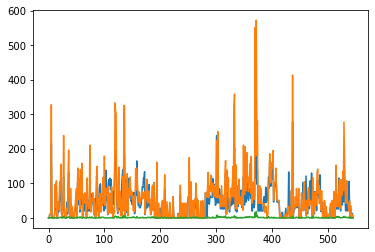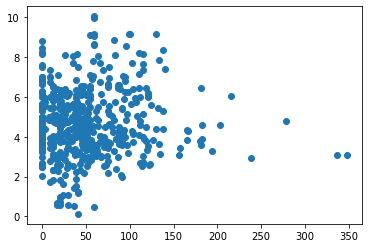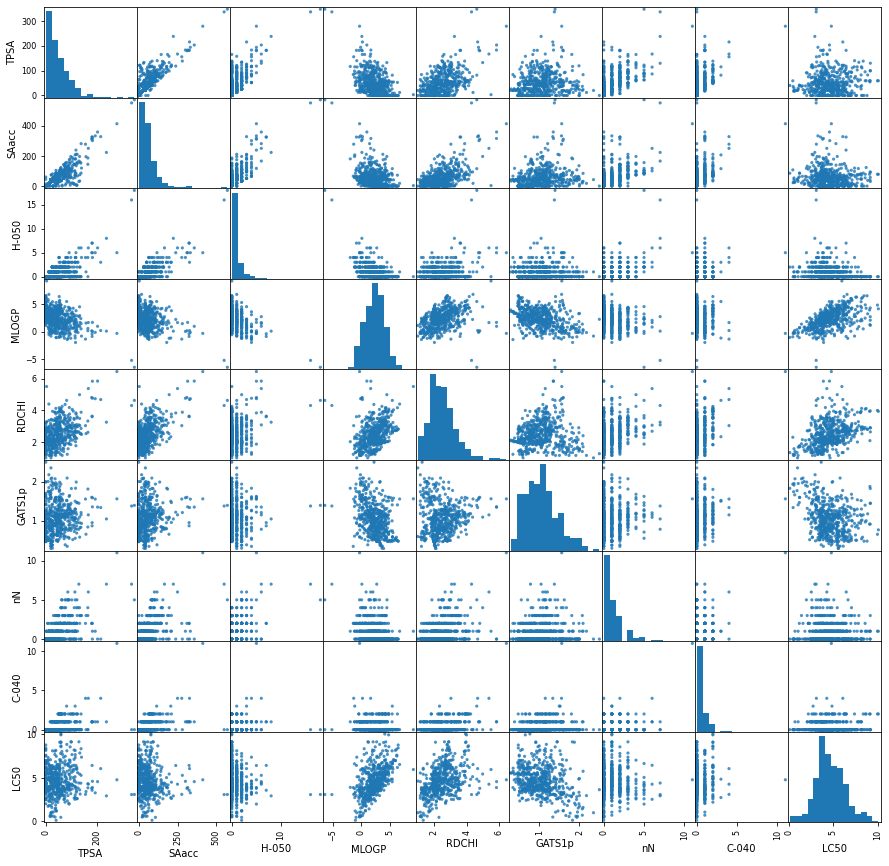# Help with A3 Assignment¶

This notebook contains code that we developed during the lecture in response to students' questions about Assignment A3.

In :
import numpy as np

In :
class NeuralNetwork():

def make_weights(self):

self.all_weights = np.zeros(22)
W1 = self.all_weights[:10].reshape(2, 5) # shape 2 x 5
W2 = self.all_weights[10:].reshape(6, 2)
self.Ws = [W1, W2]

def initialize_weights(self):

# W1[:] = np.random.uniform(-1, 1, size=(2, 5)) / np.sqrt(2)
W = self.Ws
W[:] = np.random.uniform(-1, 1, size=(2, 5)) / np.sqrt(2)

In :
nnet = NeuralNetwork()

In :
nnet.make_weights()

In :
nnet.all_weights

Out:
array([0., 0., 0., 0., 0., 0., 0., 0., 0., 0., 0., 0., 0., 0., 0., 0., 0.,
0., 0., 0., 0., 0.])
In :
nnet.Ws

Out:
[array([[0., 0., 0., 0., 0.],
[0., 0., 0., 0., 0.]]),
array([[0., 0.],
[0., 0.],
[0., 0.],
[0., 0.],
[0., 0.],
[0., 0.]])]
In :
nnet.Ws[1, 1] = 20

In :
nnet.Ws

Out:
[array([[ 0.,  0.,  0.,  0.,  0.],
[ 0., 20.,  0.,  0.,  0.]]),
array([[0., 0.],
[0., 0.],
[0., 0.],
[0., 0.],
[0., 0.],
[0., 0.]])]
In :
nnet.all_weights

Out:
array([ 0.,  0.,  0.,  0.,  0.,  0., 20.,  0.,  0.,  0.,  0.,  0.,  0.,
0.,  0.,  0.,  0.,  0.,  0.,  0.,  0.,  0.])
In :
nnet.initialize_weights()

In :
nnet.all_weights

Out:
array([-0.1535123 ,  0.31137131,  0.44323087,  0.4013435 , -0.36696818,
-0.3703361 , -0.30197005, -0.22070137, -0.53431622,  0.49782598,
0.        ,  0.        ,  0.        ,  0.        ,  0.        ,
0.        ,  0.        ,  0.        ,  0.        ,  0.        ,
0.        ,  0.        ])

In [ ]:
import optimizers as opt

# def train(self, X, T, .....) function

# deal with standardization, resulting in Xst, Tst

optimizer = opt.Optimizers(self.all_weights)
# assuming   def mse(self, X, T):
# assuming   def backward(self, X, T):

def error_convert(mse_st):
# Unstandardize the error  mean((Tst - Yst)**2)
# by doing  mean((T - Y)**2)
# Since Tst = (T - Tmean) / Tstd
#  then   T = Tst * Tstd + Tmean
# So unstandardize mse_st by  mean((Tst * Tstd + Tmean  -  (Yst * Tstd + Tmean)) ** 2)
#                         by  mean((Tst * Tstd + Tmean  -  Yst * Tstd - Tmean) ** 2)
#                         by  mean((Tst * Tstd -  Yst * Tstd) ** 2)
#                         by  mean( ((Tst -  Yst) * Tstd) ** 2)
#                         by  mean((Tst - Yst)**2  Tstd**2)
#  Now, with sqrt          sqrt(mean((Tst - Yst)**2  Tstd**2))
#               or          sqrt(mean((Tst - Yst)**2)  * Tstd

return np.sqrt(mse_st) * self.stand_params['Tstd']

if method == 'sgd':
optimizer = opt.Optimizers(self.all_weights).sgd
...

self.error_trace = optimizer(self.mse, self.backward, [Xst, Tst], 1000, 0.01,
error_convert_f=error_convert)


Does use() call forward() or not?

Answer: It doesn't have to. forward() deals with standardized variables. use() does not. But use() can call forward() after standardizing X, then unstandardize output Y before returning it.

You, or your user, just has to know NeuralNetwork, train, use functions. Knows nothing about standardization.

In :
!head qsar_aquatic_toxicity.csv




In :
import numpy as np
import pandas as pd
import matplotlib.pyplot as plt

In :
!wc qsar_aquatic_toxicity.csv

  546   546 22903 qsar_aquatic_toxicity.csv

In :
datadf = pd.read_csv('qsar_aquatic_toxicity.csv')

In :
datadf

Out:
0;0;0;2.419;1.225;0.667;0;0;3.740
0 0;0;0;2.638;1.401;0.632;0;0;4.330
1 9.23;11;0;5.799;2.93;0.486;0;0;7.019
2 9.23;11;0;5.453;2.887;0.495;0;0;6.723
3 9.23;11;0;4.068;2.758;0.695;0;0;5.979
4 215.34;327.629;3;0.189;4.677;1.333;0;4;6.064
... ...
540 24.06;35.776;2;3.326;2.837;0.849;2;0;4.651
541 9.23;11;0;3.275;2.727;0.874;0;0;3.953
542 0;0;0;5.165;3.111;0.732;0;0;6.219
543 13.14;9.507;0;2.859;2.614;0.827;0;0;4.995
544 0;0;0;2.255;1.8;0.917;0;0;2.480

545 rows × 1 columns

In :
datadf = pd.read_csv('qsar_aquatic_toxicity.csv', delimiter=';')

Out:
0 0.1 0.2 2.419 1.225 0.667 0.3 0.4 3.740
0 0.00 0.000 0 2.638 1.401 0.632 0 0 4.330
1 9.23 11.000 0 5.799 2.930 0.486 0 0 7.019
2 9.23 11.000 0 5.453 2.887 0.495 0 0 6.723
3 9.23 11.000 0 4.068 2.758 0.695 0 0 5.979
4 215.34 327.629 3 0.189 4.677 1.333 0 4 6.064
... ... ... ... ... ... ... ... ... ...
540 24.06 35.776 2 3.326 2.837 0.849 2 0 4.651
541 9.23 11.000 0 3.275 2.727 0.874 0 0 3.953
542 0.00 0.000 0 5.165 3.111 0.732 0 0 6.219
543 13.14 9.507 0 2.859 2.614 0.827 0 0 4.995
544 0.00 0.000 0 2.255 1.800 0.917 0 0 2.480

545 rows × 9 columns

In :
datadf = pd.read_csv('qsar_aquatic_toxicity.csv', delimiter=';', header=None)

Out:
0 1 2 3 4 5 6 7 8
0 0.00 0.000 0 2.419 1.225 0.667 0 0 3.740
1 0.00 0.000 0 2.638 1.401 0.632 0 0 4.330
2 9.23 11.000 0 5.799 2.930 0.486 0 0 7.019
3 9.23 11.000 0 5.453 2.887 0.495 0 0 6.723
4 9.23 11.000 0 4.068 2.758 0.695 0 0 5.979
... ... ... ... ... ... ... ... ... ...
541 24.06 35.776 2 3.326 2.837 0.849 2 0 4.651
542 9.23 11.000 0 3.275 2.727 0.874 0 0 3.953
543 0.00 0.000 0 5.165 3.111 0.732 0 0 6.219
544 13.14 9.507 0 2.859 2.614 0.827 0 0 4.995
545 0.00 0.000 0 2.255 1.800 0.917 0 0 2.480

546 rows × 9 columns

In :
names = ['TPSA', 'SAacc', 'H-050', 'MLOGP', 'RDCHI', 'GATS1p', 'nN', 'C-040', 'LC50']
names

Out:
['TPSA', 'SAacc', 'H-050', 'MLOGP', 'RDCHI', 'GATS1p', 'nN', 'C-040', 'LC50']
In :
datadf.columns

Out:
Int64Index([0, 1, 2, 3, 4, 5, 6, 7, 8], dtype='int64')
In :
datadf.columns = names

Out:
Index(['TPSA', 'SAacc', 'H-050', 'MLOGP', 'RDCHI', 'GATS1p', 'nN', 'C-040',
'LC50'],
dtype='object')
In :
datadf

Out:
TPSA SAacc H-050 MLOGP RDCHI GATS1p nN C-040 LC50
0 0.00 0.000 0 2.419 1.225 0.667 0 0 3.740
1 0.00 0.000 0 2.638 1.401 0.632 0 0 4.330
2 9.23 11.000 0 5.799 2.930 0.486 0 0 7.019
3 9.23 11.000 0 5.453 2.887 0.495 0 0 6.723
4 9.23 11.000 0 4.068 2.758 0.695 0 0 5.979
... ... ... ... ... ... ... ... ... ...
541 24.06 35.776 2 3.326 2.837 0.849 2 0 4.651
542 9.23 11.000 0 3.275 2.727 0.874 0 0 3.953
543 0.00 0.000 0 5.165 3.111 0.732 0 0 6.219
544 13.14 9.507 0 2.859 2.614 0.827 0 0 4.995
545 0.00 0.000 0 2.255 1.800 0.917 0 0 2.480

546 rows × 9 columns

In :
data = datadf.values
data

Out:
array([[ 0.   ,  0.   ,  0.   , ...,  0.   ,  0.   ,  3.74 ],
[ 0.   ,  0.   ,  0.   , ...,  0.   ,  0.   ,  4.33 ],
[ 9.23 , 11.   ,  0.   , ...,  0.   ,  0.   ,  7.019],
...,
[ 0.   ,  0.   ,  0.   , ...,  0.   ,  0.   ,  6.219],
[13.14 ,  9.507,  0.   , ...,  0.   ,  0.   ,  4.995],
[ 0.   ,  0.   ,  0.   , ...,  0.   ,  0.   ,  2.48 ]])
In :
data.dtype

Out:
dtype('float64')
In :
plt.plot(data[:, 0:3])

Out:
[<matplotlib.lines.Line2D at 0x7f6e3f6fc400>,
<matplotlib.lines.Line2D at 0x7f6e3f6fc4f0>,
<matplotlib.lines.Line2D at 0x7f6e3f6fc5b0>]In :
datadf.describe()

Out:
TPSA SAacc H-050 MLOGP RDCHI GATS1p nN C-040 LC50
count 546.000000 546.000000 546.000000 546.000000 546.000000 546.000000 546.000000 546.000000 546.000000
mean 48.472930 58.869018 0.937729 2.313493 2.492299 1.046264 1.003663 0.353480 4.658421
std 46.763983 68.166554 1.618632 1.741797 0.811004 0.403677 1.397240 0.806827 1.665215
min 0.000000 0.000000 0.000000 -6.446000 1.000000 0.281000 0.000000 0.000000 0.122000
25% 15.790000 11.000000 0.000000 1.232500 1.975000 0.737000 0.000000 0.000000 3.601500
50% 40.460000 42.683000 0.000000 2.273500 2.344000 1.020500 1.000000 0.000000 4.516000
75% 70.022500 77.492750 1.000000 3.392750 2.911000 1.266500 2.000000 0.000000 5.607500
max 347.320000 571.952000 18.000000 9.148000 6.439000 2.500000 11.000000 11.000000 10.047000
In :
plt.plot(data[:, 0] , data[:, -1], 'o')

Out:
[<matplotlib.lines.Line2D at 0x7f6e3f543190>]In :
pd.plotting.scatter_matrix(datadf, figsize=(15, 15), marker='o', hist_kwds={'bins': 15},
s=10, alpha=0.8);In :
import seaborn as sns